Friday, December 1, 2023
HomeAIWhat is Clustering?

# What is Clustering?

Clustering is a machine learning technique widely applied in society, such as customer data analysis and product recommendation systems.

Various classification algorithms have long been devised in the fields of statistics and AI, and clustering can be said to be one of the most successful examples.

However, some readers may not be familiar with clustering.

Therefore, this time, we will explain in detail the outline, merits and demerits of ” clustering “, which is one of the representative methods of machine learning, and application examples.

• What is Clustering?
• Difference from classification
• hierarchical clustering
• non-hierarchical clustering
• Comparing Hierarchical and Non-Hierarchical Clustering
• Curse of Dimension
• Use cases for clustering
• Company and sales strategy planning
• Image classification/sound classification
• summary

## What is Clustering?

Clustering is a machine learning technique that divides data into groups based on similarity between data .

Each classified subset is called a “cluster”. Clustering is sometimes called cluster analysis or cluster analysis.

Clustering is classified as ” unsupervised learning ” among machine learning methods . Since there is no need to assign features (labeling) to each data, it is possible to automatically classify large amounts of big data.

1. Difference between classification and clustering
2. hierarchical clustering
3. non-hierarchical clustering

We will discuss this from the above three perspectives.

### Difference from classification

“Classification” and ” clustering “, which are often heard in machine learning, are sometimes confused because they are both methods of classifying groups of data.

Clustering is unsupervised learning whereas classification (classification) is supervised learning.

The difference can be represented as shown in the figure below.

In this way, the problem with clustering is how to group uncharacterized data. The methods are roughly divided into two types: hierarchical clustering and non-hierarchical clustering.

### hierarchical clustering

A basic hierarchical clustering method is to sequentially cluster the data that are closest to a given point and group them into a single structure as a hierarchy. The structure is, to put it bluntly, a tree diagram.

Now, let’s actually follow the hierarchical clustering method using the data from A to F below.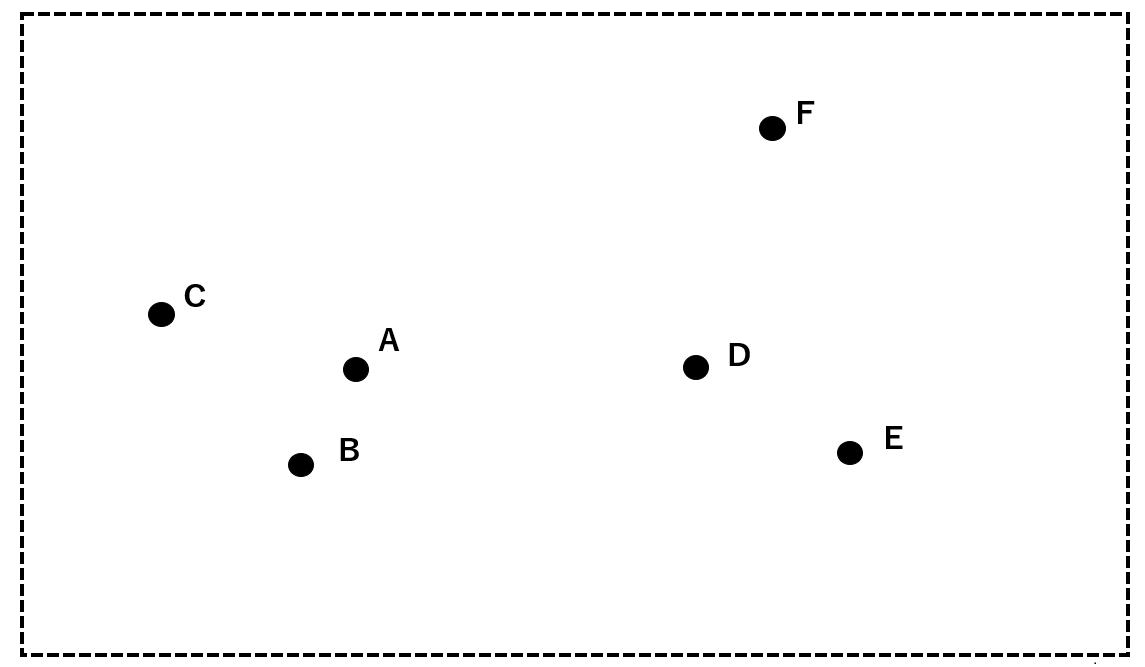First, group A and B, which are closest to each other, into a single cluster.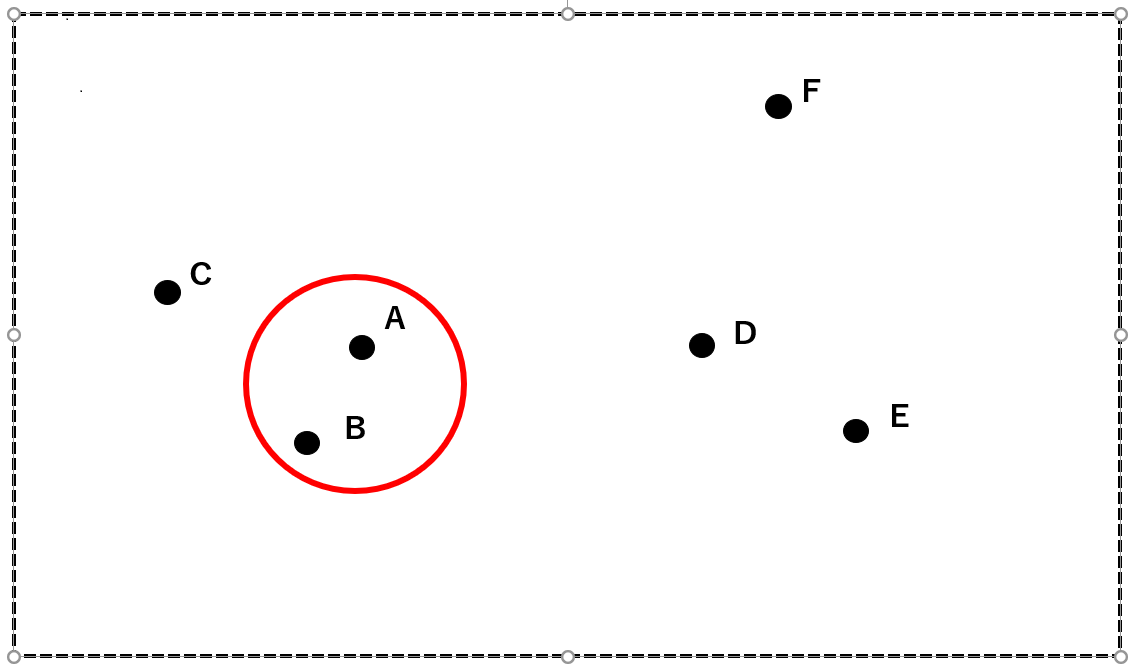Next, the cluster consisting of A and B (red) and the closest two of C, D, E, and F are combined into one cluster. Here, let D and E be clusters (blue).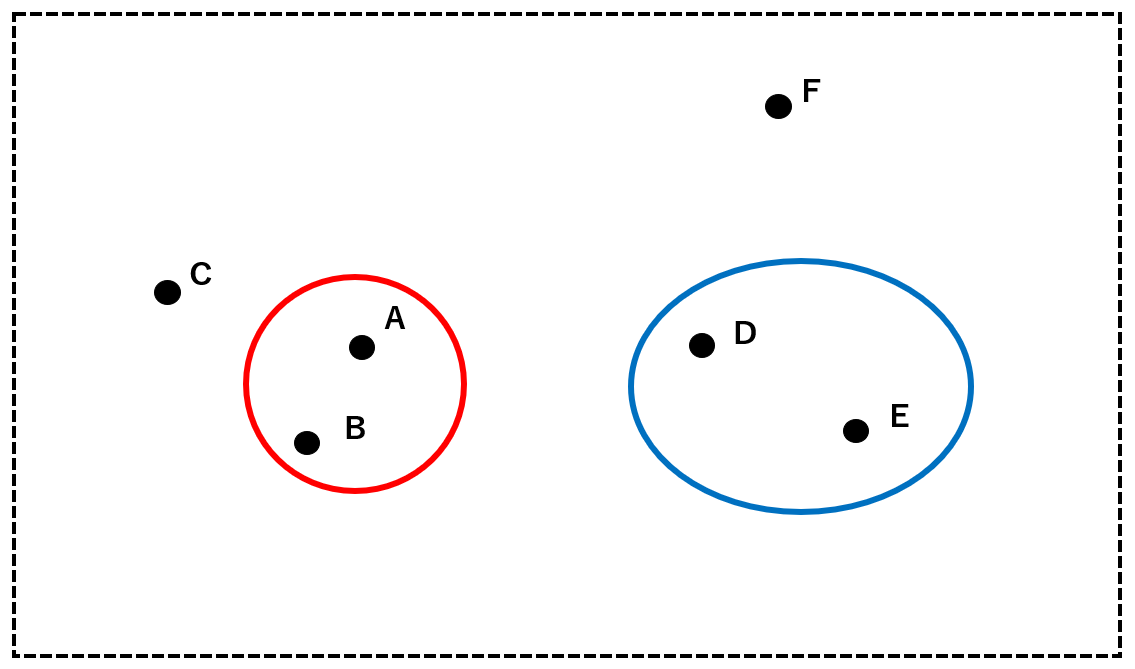Next, cluster (red), cluster (blue), C, and F, which are closest to each other, are grouped together. Here we combine the cluster (red) and C into a cluster (green). In the same way, cluster (blue) and F are grouped into cluster (yellow).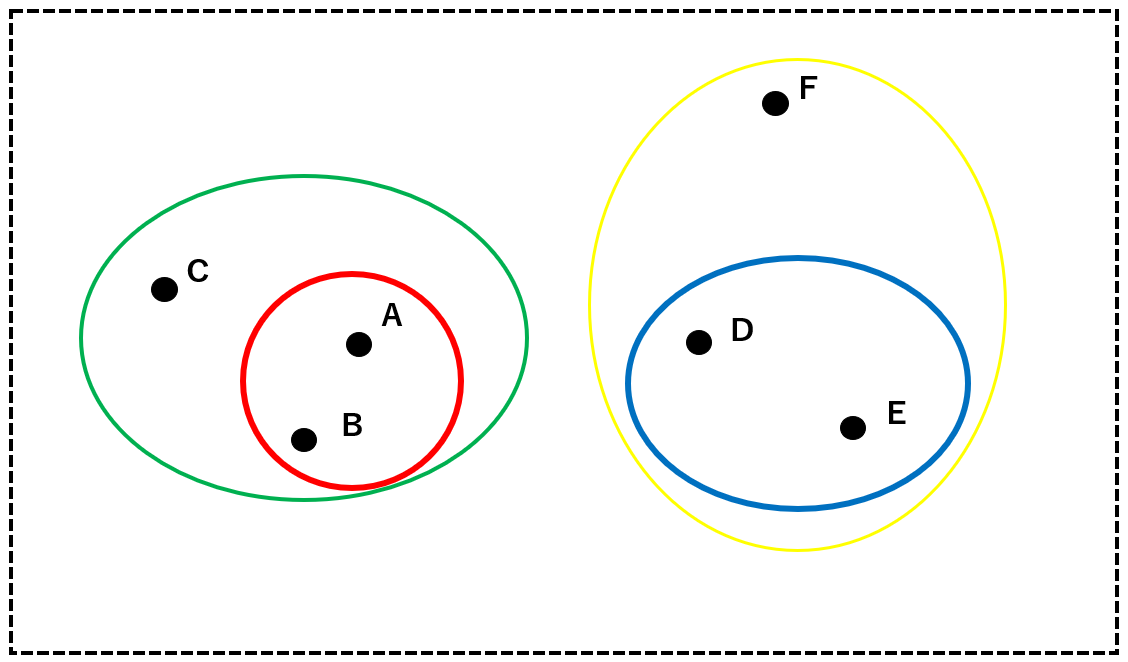Finally, the analysis ends with a cluster (purple) that subsumes all clusters.Representing these structures hierarchically results in a tree diagram like the one below.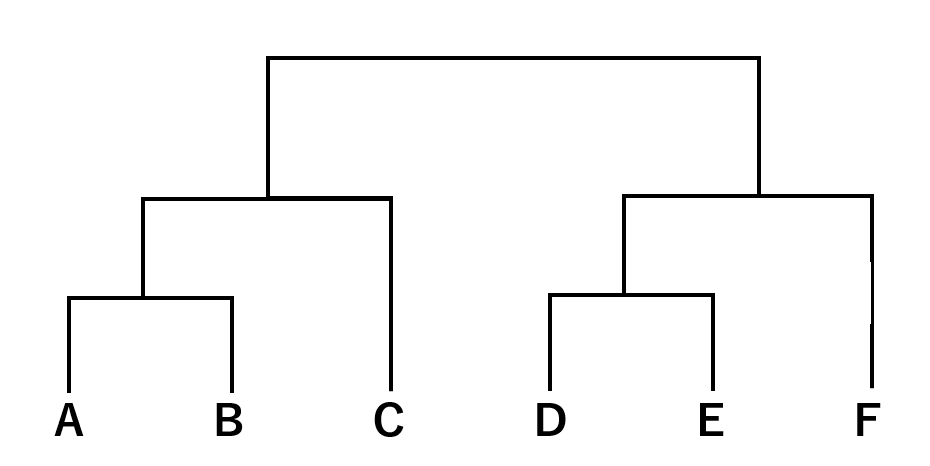In this example, we proceeded without strictly defining “how close two parties are”, but there are several variations on how to determine the “inter-cluster distance”.

#### Group average method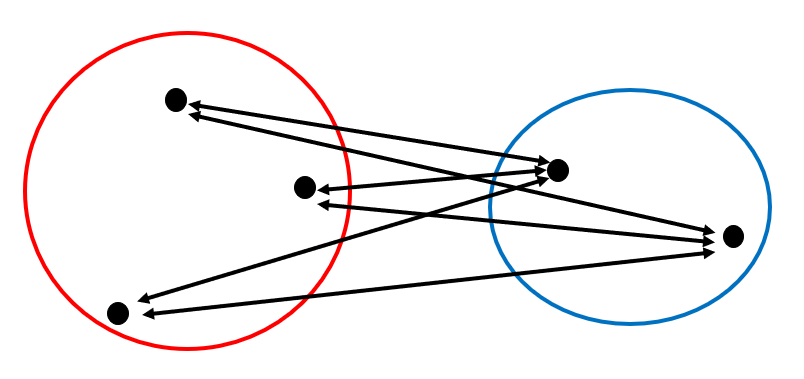The group average method is a method in which the average of the distances between samples that make up a cluster is taken as the distance between clusters. It is strong against outliers and abnormal values, which are factors that degrade accuracy, and analysis is stable. Therefore, it is a method generally used as a method to determine the distance between clusters.

#### Longest distance method/shortest distance methodAlternatively, simply, the method of taking the distance between the furthest samples that make up each cluster as the inter-sample distance is called the longest distance method. In addition, the method of taking the distance between the closest objects as the inter-sample distance is called the shortest distance method.

Although these methods have a small amount of calculation, they each have disadvantages, so they are not often used in practice.

#### Ward Law

Ward’s method is a method of creating a new cluster that minimizes the difference between the sum of two clusters for the distance between all samples and centroids in the cluster before combination and the variance in the cluster after combination. say. This method has excellent accuracy, but the computational complexity is very large.

### non-hierarchical clustering

Non-hierarchical clustering, as the name suggests, is a method of classifying data into clusters without organizing it into a hierarchical structure (such as a tree diagram).

Hierarchical clustering described above is said to be unsuitable for big data analysis because all possible combinations must be calculated, resulting in an enormous amount of computation.

Non-hierarchical clustering involves artificially predetermining the number of clusters to classify before starting the computational analysis. The most representative method is the k-means method.

#### k-means method

In the k-means method, k centroid points (nuclei) are randomly determined for the sample group.Calculate the distance between every sample and k nuclei and classify each sample to the closest nucleus.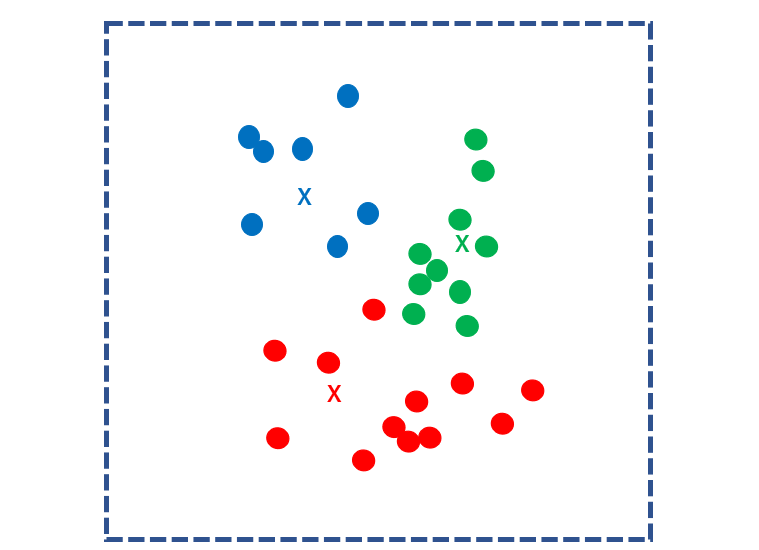Next, find the centroid point for each cluster and make it the new k kernels. Again calculate the distance between every sample and k nuclei and classify each sample to the nearest nucleus.Repeat this step until the center of mass no longer moves. The calculation ends when the centroid point is no longer updated.

Among many machine learning methods, clustering is classical yet has relatively stable performance. The advantages and disadvantages of clustering are discussed below.

### Comparing Hierarchical and Non-Hierarchical Clustering

 hierarchical clustering non-hierarchical clustering computational complexity many Few number of clusters freedom must be specified in advance unique problem computationally expensive Initial value dependent

As mentioned above, hierarchical clustering does not require the number of clusters to be determined in advance, but the computational complexity is enormous. Therefore, hierarchical clustering is not suitable for big data processing.

While non-hierarchical clustering is less computationally expensive, we have to artificially specify the number of clusters in advance.

Also, with the k-means method, the results can vary greatly depending on the initial kernel (center of gravity), making it difficult to obtain stable results. This phenomenon is called “initial value dependence”.

### Curse of Dimension

One of the problems with clustering is the “curse of dimensionality”.

When calculating data with many dimensions (number of explanatory variables), the amount of calculation increases exponentially with the number of dimensions.

Overfitting occurs due to the “curse of dimensionality” when clustering data that is not distributed that much in a huge number of dimensions (data with relatively strong correlations in each dimension) or when clustering data with a small amount of data. more likely to.

The curse of dimensionality, such as k-nearest neighbors, is a typical problem not only in clustering but also in many other methods. Overfitting can occur in these analyses, as the number of dimensions increases in the process of computation. The same problem has also been pointed out in the field of deep learning.

## Use cases for clustering

Clustering is widely used in non-technical fields such as marketing and product appeal because the results can be visualized in an easy-to-understand manner using tree diagrams and color coding.

### Company and sales strategy planning

For example, by analyzing customer data, you can analyze groups of customers with similar attributes. By making strategies for each group and appealing products and information, more efficient marketing is possible.

Alternatively, you can determine which group (cluster) your company’s customers belong to by comparing it with other companies’ customer data. With this data, you can strengthen your company’s branding.

### Image classification/sound classification

By clustering big data consisting of image data and audio data, data with similar tendencies can be classified.

For example, by classifying music with relatively similar rhythms and tonalities, it is possible to create clusters with attributes such as “music to listen to at night” and “music to listen to when you want to lift your mood.”

## summary

Clustering , especially non-hierarchical clustering such as k-means, is a simple algorithm that can handle complex classification problems.

On the other hand, it has a typical AI problem represented by the “curse of dimensionality”.

Attempts to overcome these clustering problems are still ongoing, and recently new methods such as “DeepCluster” and “SeLa” have attracted attention. Clustering is expected to further develop in the future.

RELATED ARTICLES